"Additional Loss Due to SWR" is in Quotes for a Reason – or – Think Twice Before Using a Web-Based Line Loss Calculator Dan Maguire, AC6LA

Online coax loss calculators that use SWR as one of the inputs certainly are convenient but can you trust the results? Alternative tools which are much more accurate are readily available.

The Problem

Most of the online calculators use the ARRL's "Additional Loss Due to SWR" formula, shown (with minor edits) below.The advantage of using this formula is that it is simple and thus easy to implement using JavaScript. That's why so many online calculators all look the same; they all use the same script code in the html of the web page. And they all copied that script directly or indirectly from the original.

The disadvantage of the ARRL formula is that the results may or may not be particularly accurate. And there's no way to know without doing more rigorous calculations.

The Solution

The first step is to use the basic "Transmission Line Equation", also called the "Telegrapher's Equation", which calculates the impedance at the input end (near end) of a line given the impedance at the load (far end, termination) and the characteristics of the line itself.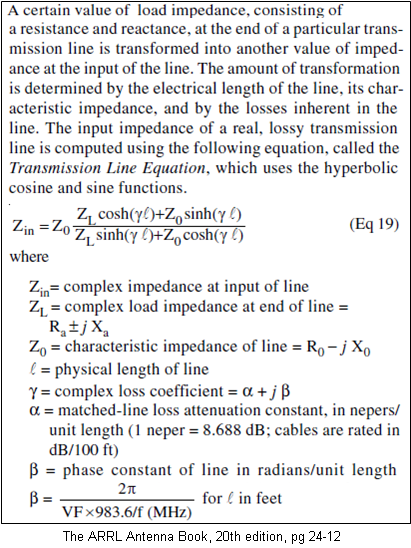Once you know the impedance at both ends of the line there are several different ways to calculate the total dB loss. The one that has been used in all AC6LA software (except JavaScript) for the past 15 years or so was described by Charles Michaels, W7XC (SK), in the November 1997 issue of QST.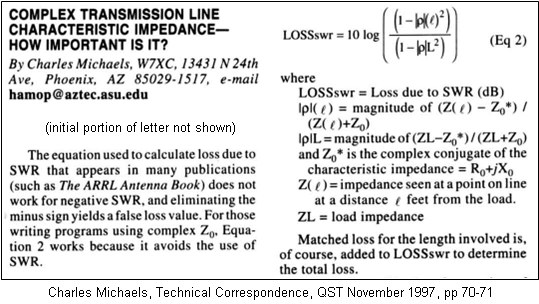Note that "LOSSswr" calculated above can be negative in some cases. That's not the same as a negative SWR which happens when the magnitude of the (normal) reflection coefficient is greater than one.

Another method was described by Steve Stearns, K6OIK, in a presentation titled "A Transmission Line Power Paradox and Its Resolution" given at Pacificon 2014. Here's page 43 from the K6OIK presentation.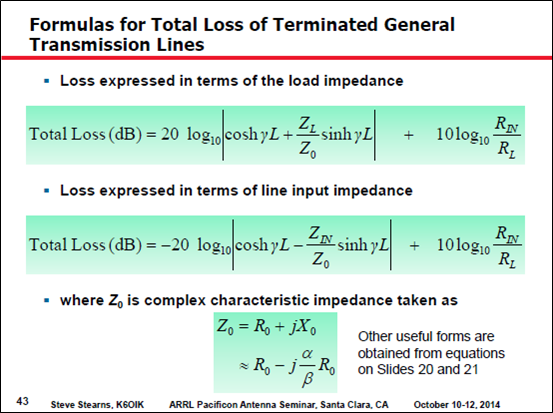The full K6OIK presentation can be downloaded here. It includes a very nice explanation of the flawed assumption in the ARRL formula and why the formula is accurate only in special cases, none of which have anything to do with the stated warning of "SWR levels less than 20:1".

Yet another method is convenient if you also want to calculate the current and voltage at either end of the line. The lower formulas below calculate the current at the load (termination) based on the current at the input; on the left using the impedance at the load, on the right using the impedance at the input.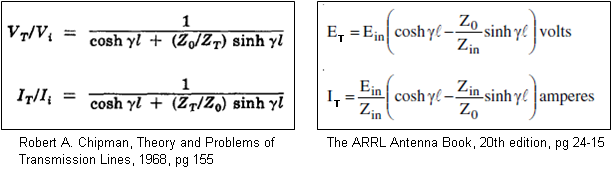With an arbitrary current, say 1 amp rms, at the input end of the line the above formulas give the current at the load (termination). Using those values you can compute the power levels at each end:

Pin = |Iin|^2 * Rin
Then the dB loss is:
Total line loss (dB) = 10 * Log10(Pin / Pload)
Still another way to compute total line loss is to use the "lossy transmission line" feature of EZNEC v. 5.0.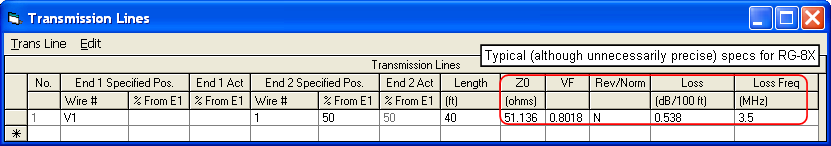EZNEC will calculate the impedance (and power) at the source, the input end of the line. EZNEC will also calculate the current at the antenna feedpoint, the load end of the line. Knowing the feedpoint current along with the feedpoint resistance you can calculate the power at the load. With Pin and Pload you can calculate total line loss in dB as shown previously.

So how do all these methods compare? Rather than using some arbitrary load impedance let's examine an 80m dipole that has been cut slightly long and is fed with RG-8X. In particular, at 3.5 MHz a 140 ft #12 wire at a height of 30 ft over average ground has a modeled feedpoint impedance of 54.52+j62.84 ohms. With that load impedance and using transmission line characteristics typical for RG-8X here is a plot of the calculated total line loss for line lengths from 5 to 200 ft.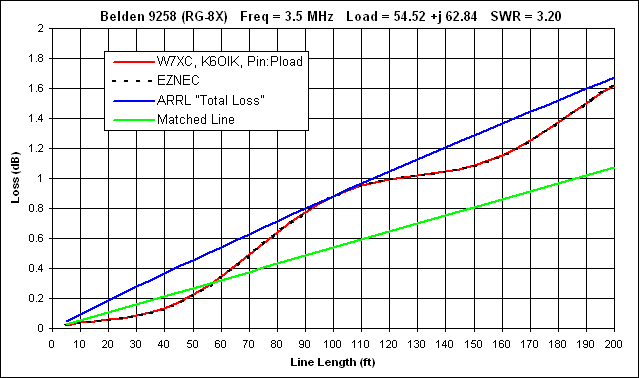The W7XC, K6OIK, and Pin:Pload formulas all produce identical results. The EZNEC "lossy transmission line" values differ from the more precise values by an average of 0.004 dB, barely detectable on the plot. On the other hand, the ARRL "Total Loss" formula is accurate only by happenstance at certain line lengths. And note that the SWR at the load is 3.20:1, not at all extreme.

Also interesting is that for this combination of load, transmission line, and frequency, at lengths below about 55 ft the total power loss is actually less than the "matched line" loss. That's a tough concept to grasp if you've always thought of "matched line loss" as being equivalent to "minimum loss". For more on this subject see Steve Stearns, K6OIK, "Loss Formulas for General Uniform Transmission Lines and Paradox 5", QEX Sep/Oct 2021, pp 18-28.

In the example above, the ARRL approximation was "too high" at most line lengths. But that is not always the case. Here is the situation if the reactance of the load is set as negative 62.84 ohms. Notice that the precise values are now on the "other side" of the ARRL loss values.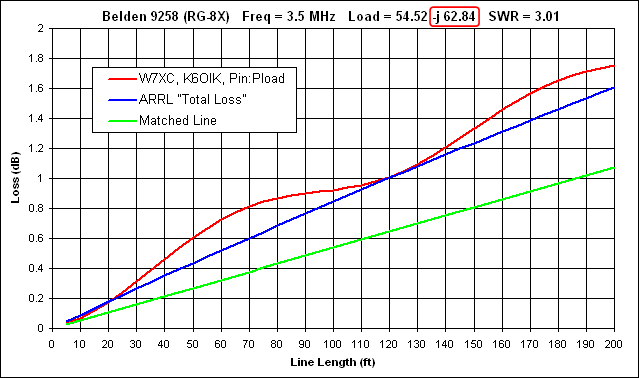Now in all fairness we need to dig a little deeper. The discrepancy between the ARRL approximation and the more accurate calculations is due to the reactive component of the line's characteristic impedance; the Xo part of Zo=Ro-jXo. If Xo is arbitrarily set to zero then the ARRL formula matches the more accurate calculations exactly.

The characteristic impedance Zo of real transmissions lines at frequencies below the GHz range always has a -jXo component, but Xo decreases in magnitude (gets closer to zero) at higher frequencies and for better quality lines with less inherent loss. So suppose we scale the slightly-long dipole up to 14 MHz and feed it with RG-213. Assuming the antenna stays at a height of 30 ft the feedpoint impedance will be 84.51+j28.50 ohms. And with that load impedance at the end of 5 to 200 ft of RG-213 here's the total line loss.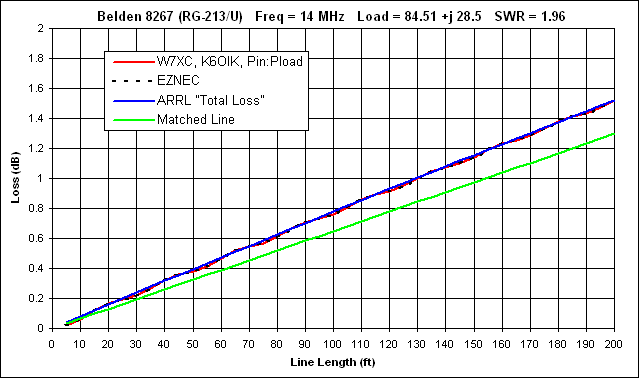Pretty close. But of course you wouldn't know that unless you did the exact calculations.

Here's another interesting example. This is the scenario described on pp 44-50 of the K6OIK presentation; an electrically very short antenna fed with Wireman 551 ladder line. The line length steps have been extended out to 500 ft, just a bit beyond one full wavelength.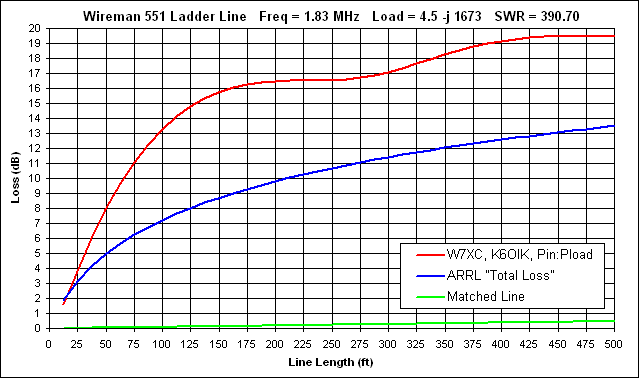Because of the extreme SWR at the load there is no expectation that the ARRL formula should be accurate. But again, the only way to get the correct loss is to do the calculations. And there are plenty of tools available to help.

The Tools

Several different tools are available which will do all the math for you. Each example shown below is for the 80m dipole at 3.5 MHz with a feedpoint (load) impedance of 54.52+j62.84 ohms fed with 40 ft of RG-8X.

First up is TLDetails. Select a line type and enter the frequency, load R/X, and line length.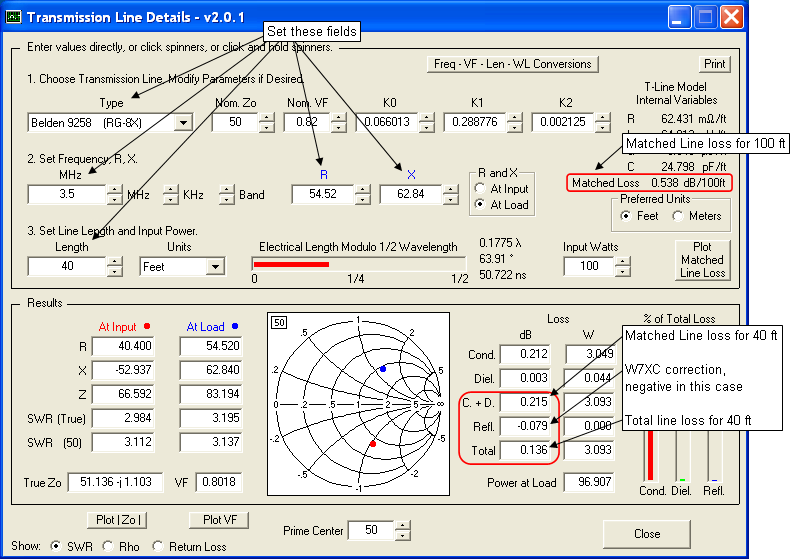Or you could use the TLW program by N6BV. The specs for RG-8X that are built-in to TLW are not quite the same as those used by TLDetails, but you can set a "User-Defined" transmission line to allow comparison with other tools. Or you could use the TLW RG-8X specs when working with the other tools. There is no "Gospel Truth" set of specifications for any given transmission line type.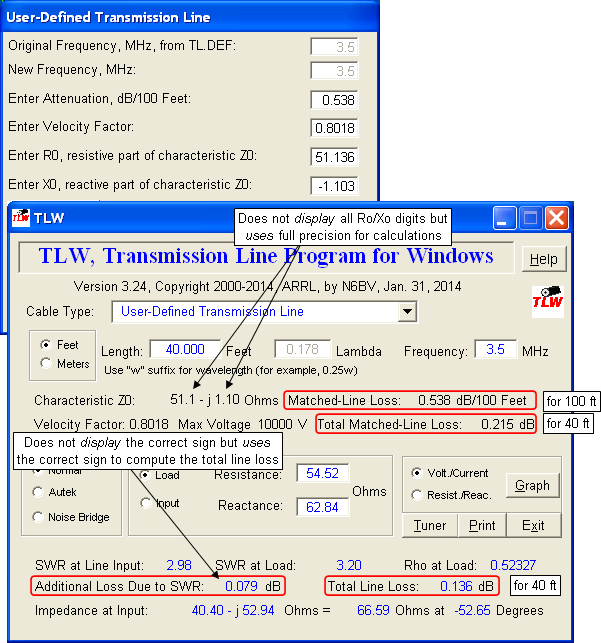Since you need to know the load impedance (the impedance at the antenna feedpoint) anyway, you can use EZNEC v. 5.0 to provide all the data required to calculate the total line loss as well. First create a model with no transmission line (source at the antenna feedpoint) and look at the "Source Data" window. Make a note of the resistance value. Then add a transmission line to the model and recalculate. Now look at the "Source Data" window again to get the power in to the transmission line. Then look at the "Currents" window to get the magnitude of the current at the antenna feedpoint, the load end of the line. Use that value along with the previously-calculated feedpoint resistance to calculate the power at the load end of the transmission line. Then use the power values to calculate the total line loss in dB.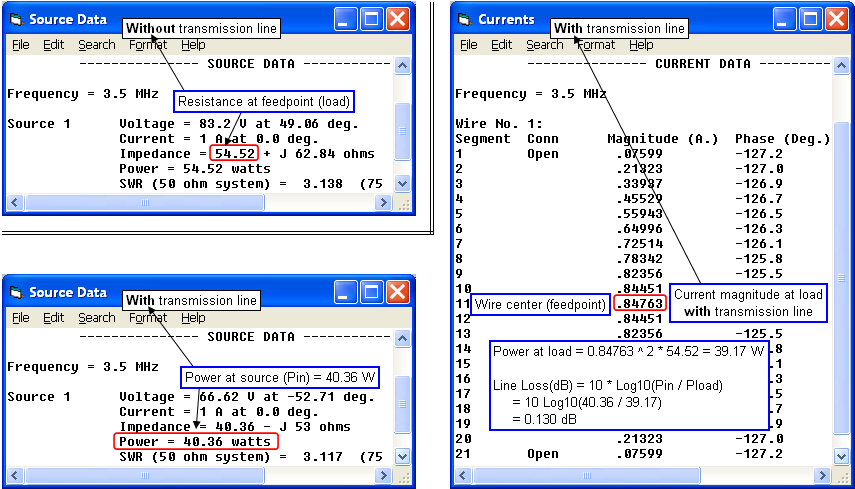Call that Method A; it's just pocket calculator math. But there's an even easier Method B. In the first model, without a transmission line, make a note of the "Slice Max Gain" as shown in the 2D Plot window. Then add the transmission line and note the new "Slice Max Gain". The difference between those two values is the power that was lost in the line. The value will be limited to two decimal places because that's the limit on gain shown by EZNEC. Don't lose any sleep over that.If you have AutoEZ you can do everything at once, plus calculations for multiple line lengths, plus let Excel do all the math. Create the wire(s) for your model, put a 1 amp current source at V1, then define a transmission line with End 1 at V1 and End 2 at the antenna feedpoint. Set the length using a variable, "=L" in the illustration below. Then click the "Set Zo, VF, and Loss" button. Select from the list of approximately 100 built-in line types or set the wire diameter and spacing for custom parallel conductor line. When you click OK the remaining fields for the transmission line will be filled in.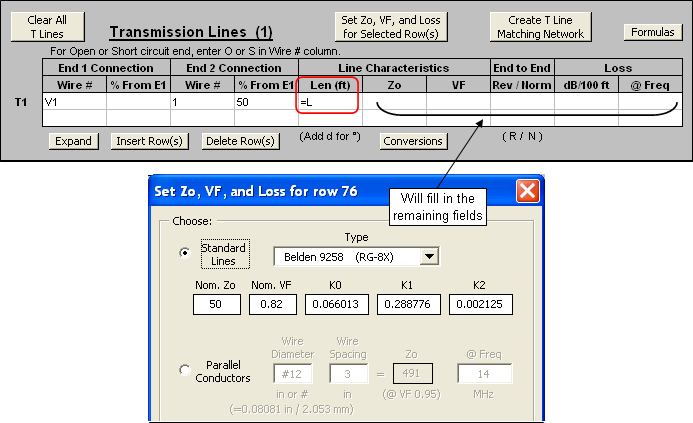On the Calculate sheet set up a series of test cases for different line lengths (variable "L") with the first value being a "virtual zero" length. You can't set the length to exactly zero but 0.001 ft is essentially the same thing. Thus the first test case is equivalent to "without transmission line" and the remaining test cases are "with transmission line" at increasing line lengths.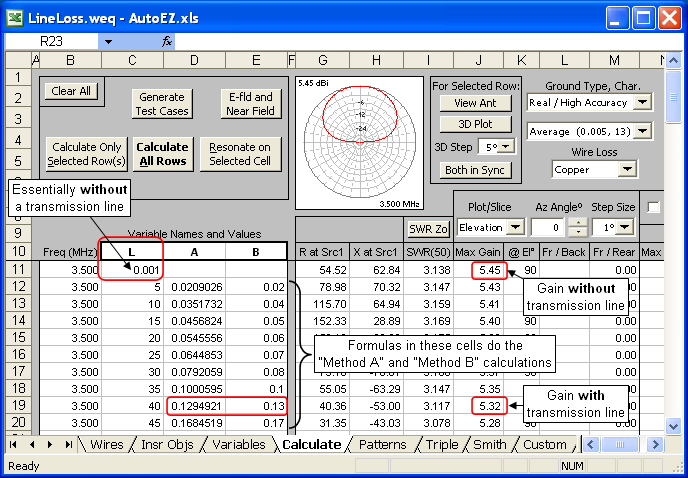Note that variable "L" is being used as an input or control variable; its value sets the length of the transmission line for any given test case row. On the other hand, variables "A" and "B" are being used as output or results variables. The cell formulas for variables "A" and "B" do the calculations described as Method A and Method B earlier. (A sample model is available showing the Method A formula although the simple subtraction of Method B is perfectly adequate.)

After you calculate all rows you can tab to the Custom sheet and plot "Variable 2" ("A") or "Variable 3" ("B") vs "Variable 1" ("L", line length).If you are not a user of EZNEC or AutoEZ you can still plot the loss for multiple line lengths as long as you have Microsoft Excel (not an Excel clone). Use the small workbook that was created for this project. It includes a library of line types with the same specifications as TLDetails and AutoEZ. All you have to do is set the frequency, choose a line type, and set the load R/X values.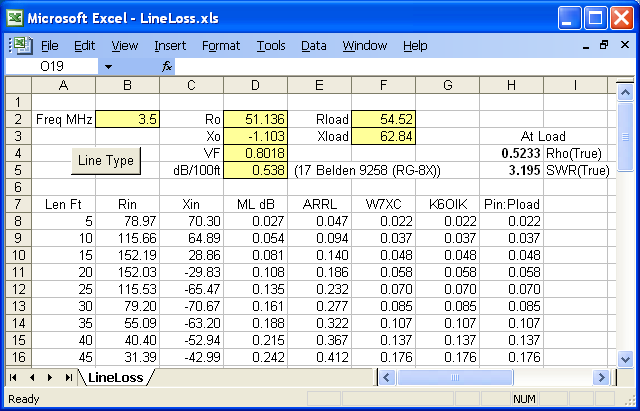If you enjoy noodling around with Excel you are more than welcome to look under the hood to see how things were done.

Appendix: Some History

A JavaScript calculator with a dropdown list of 47 different transmission lines and an input box for SWR at the load end of the line was developed by the author in 2001 as part of the web page documentation for an Excel application called XLZIZL. This is what the original looked like.Both XLZIZL and the calculator web page were withdrawn several years later. However, the JavaScript source code has been copied and re-copied many times over. A quick Google search reveals at least 30 different sites that now use exactly the same script code along with exactly the same dropdown list of line types. The copies are found on personal amateur radio sites, radio club sites, commercial sites, and academic sites. A few are even in different languages.The author is indeed flattered by all the imitators. But please keep in mind that there are other tools available which are both more comprehensive and more accurate.

Dan Maguire, AC6LA
April 2015

Home